# Examples

## Cubic diffusion with unimodal transition density

Consider a diffusion process with dynamics given by the SDE: $dX_t = -X_t^3dt + dB_t.$ Here, the drift term $$\mu(X_t,t) = - X_t^3$$ operates as a mean-reverting term, pulling the process towards the origin. In order to calculate the transitional density of the diffusion of the process: $X_t = X_s - \int_s^tX_u^3 du +\int_s^t dB_u,$ we can use the method of lines in order to solve the corresponding Kolmogorov equation. Using the MOL.density() function, we can set up and solve a system of ODEs which approximate the transitional density on a finite lattice of the state-space. In R:

library("DiffusionRimp")
# Set the parameters of the problem:
Xs   <- 1
s    <- 0
t    <- 5
lims <- c(-3, 3)
delt <- 0.0025
nodes<- 101

# Define drift and diffusion terms:
mu  <- function(X,t){-X^3}
sig <- function(X,t){1}
# Approximate the transitional density:
res <- MOL.density(Xs, s, t, lims, nodes, delt)

# Plot the density:
persp(res$Xt, res$time, pmin(res$density, 1), col = 'white', xlab = 'State (X_t)',ylab='Time (t)', zlab='Density f(X_t|X_s)', border = NA, shade = 0.5, theta = 145)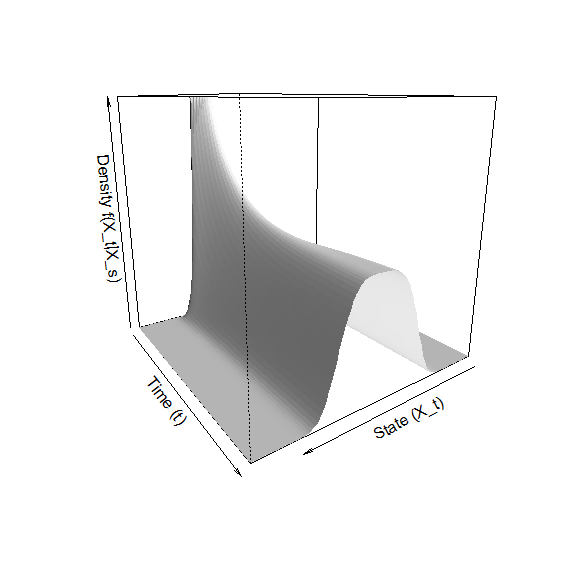Here, lims <- c(-3, 3) sets the limits of the lattice whilst nodes<- 101 breaks up the strip $$[-3,3]$$ into 101 equispaced points in order to construct the lattice. The parameters s <- 0 and t <- 5 give the transition horizon on which to approximate the transitional density whilst delt gives the step size for the numerical scheme which solves the ODE approximation to the transitional density. Note that, although the transitional density appears superficially similar to the Normal distribution, the density function becomes quite flat at the mode of the density as time progresses. ## Cubic diffusion with multimodal transition density Consider now a diffusion process with dynamics given by the SDE: $dX_t =X_t(1 -X_t^2)dt + dB_t.$ In comparison to the previous example, this diffusion has an additional term in the drift coefficient. In this case the drift function has 3 distinct zeroes with two reversion loci at $$1$$ and $$-1$$. Consequently, the transitional density has the property that it has a bimodal distribution. This model is also known as the “Double-Well Potential” (Aït-Sahalia 1999). # Define drift and diffusion terms: mu <- function(X,t){X-X^3} sig <- function(X,t){1} # Approximate the transitional density: res <- MOL.density(Xs, s, t, lims, nodes, delt) # Plot the density: persp(res$Xt, res$time, pmin(res$density, 1), col = 'white', xlab = 'State (X_t)',ylab='Time (t)',
zlab='Density f(X_t|X_s)', border = NA, shade = 0.5, theta = 145)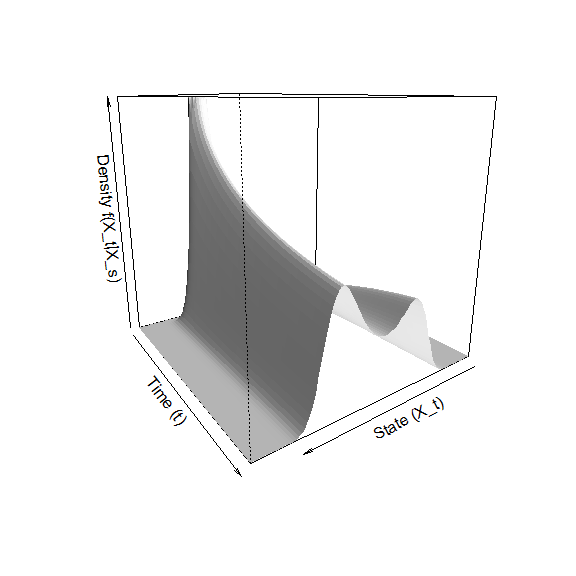## Time-inhomogenous Double-Well model

Using the method of lines it is possible to analyse generalisations of existing models such as the Double-Well model. For example, under time-inhomogeneous drift and diffusion coefficients: $dX_t =X_t((1+\cos(2\pi t)) -X_t^2)dt + (1+0.25\sin(3 \pi t))dB_t$ the transitional density reflects similar time-inhomogeneous features:

# Define drift and diffusion terms:
mu  <- function(X,t){X*((1+cos(2*pi*t))-X^2)}
sig <- function(X,t){(1+0.25*sin(3*pi*t))}
# Approximate the transitional density:
res <- MOL.density(1, 0, 5, c(-3, 3), 101, 0.0025)

# Plot the density:
for(i in 0:1)
{
main = paste0('Transition Density \n (t = ', restime[i],')'), xlab='Xt',ylab='Yt') }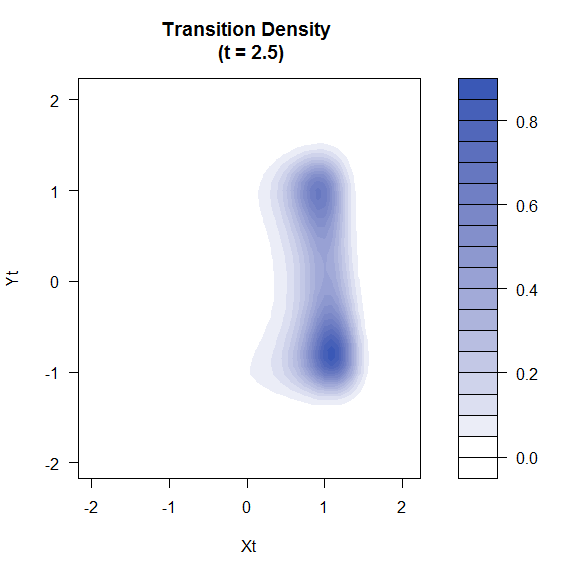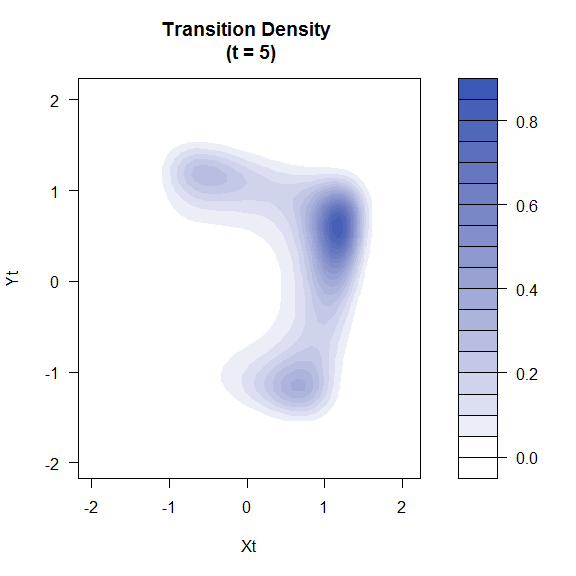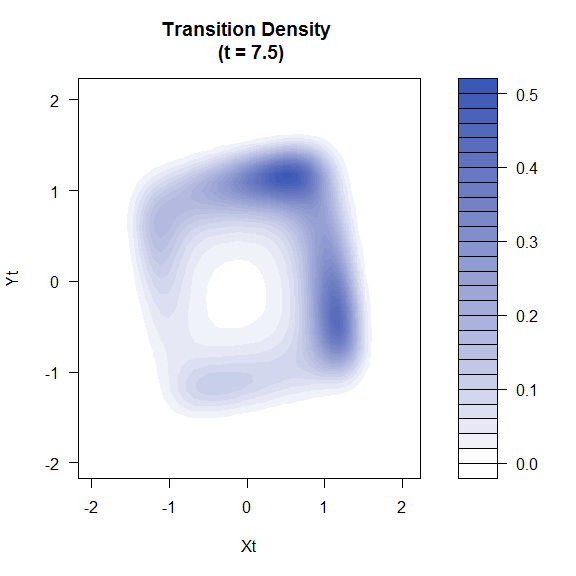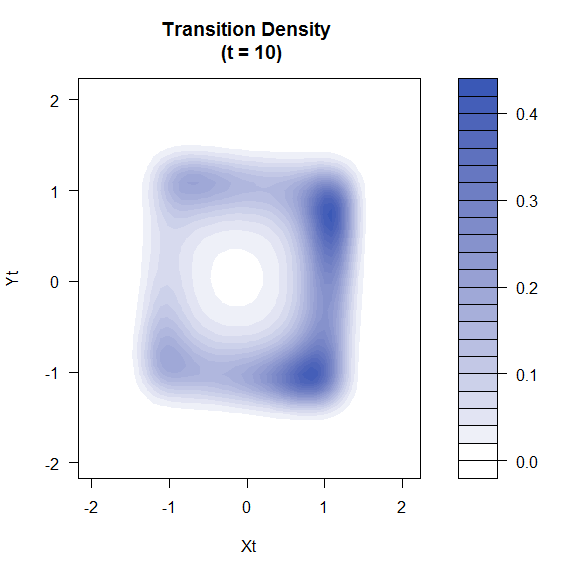## A more complicated multi-modal model Consider a diffusion process with dynamics governed by the SDE: \begin{aligned} dX_t &=[-Y_t\sin(X_t\pi)]dt + 0.5dB_t^{(1)}\\ dY_t &=[-X_t\sin(Y_t\pi)]dt + 0.5dB_t^{(2)}\\ \end{aligned} subject to the initial conditions $$(X_0,Y_0) = (0,0)$$. Solving for the transitional density on the time horizon $$[0,2.5]$$ we can see some interesting dynamics. In R: # Define drift and diffusion terms: mu1 <- function(X,Y,t){-Y*sin(X*pi)} mu2 <- function(X,Y,t){-X*sin(Y*pi)} sig11 <- function(X,Y,t){0.5} sig22 <- function(X,Y,t){0.5} # Parameters of the problem: X0 <- 0 # Initial X-coordinate Y0 <- 0 # Initial Y-coordinate s <- 0 # Starting time t <- 2.5 # Final horizon time Xlim <- c(-3,3) # Lattice endpoints in X dim Ylim <- c(-3,3) # Lattice endpoints in Y dim Nodes <- 121 # How many nodes per dimension (incl. ends) delt <- 1/200 # Step size # Run the Method of Lines: res <- BiMOL.density(X0, Y0, s, t, Xlim, Ylim, Nodes, delt) time.sequence <- c(0.5,1,1.5,2,2.5) k = 0 for(i in time.sequence) { k = k+1 persp(resXt,res$Yt,res$density[,,i/delt],col='white',xlab='State (X_t)',ylab='State (Y_t)',zlab='Density',border=NA,shade=0.5,theta=145+180*k/5)
}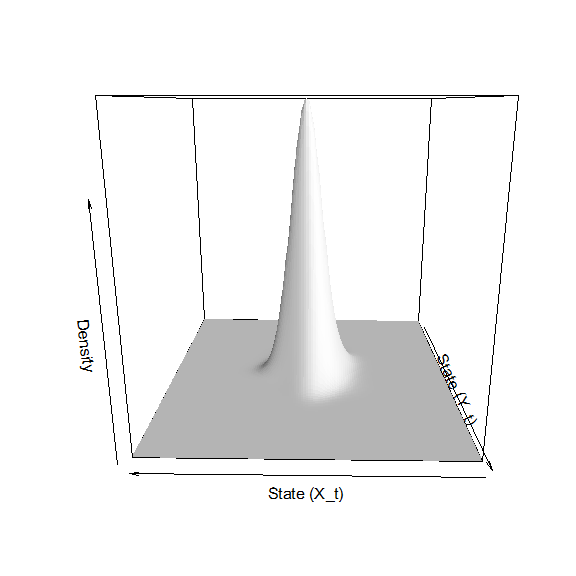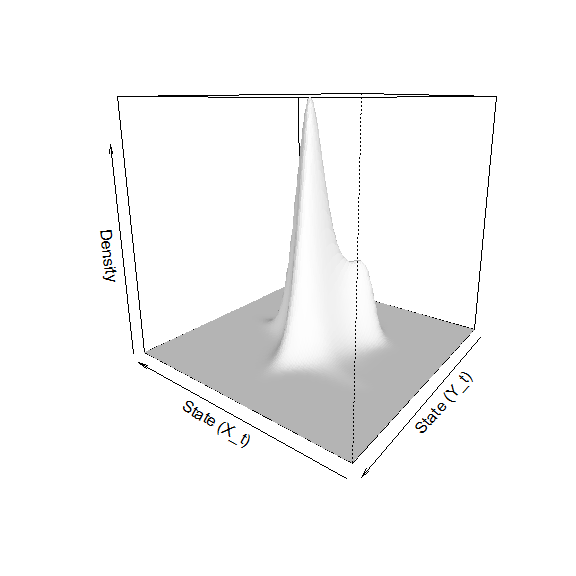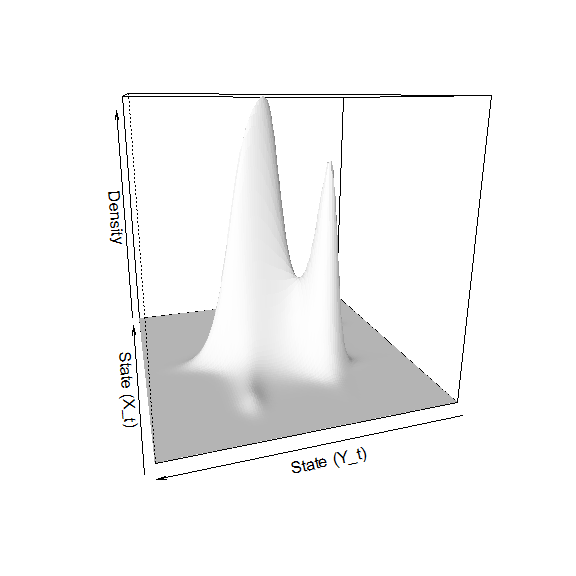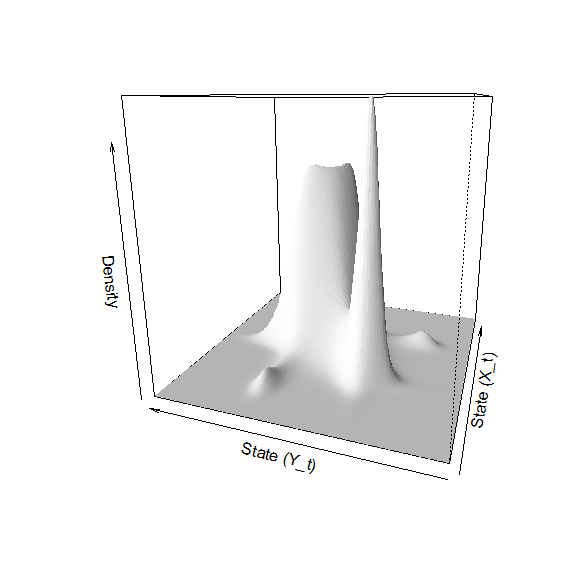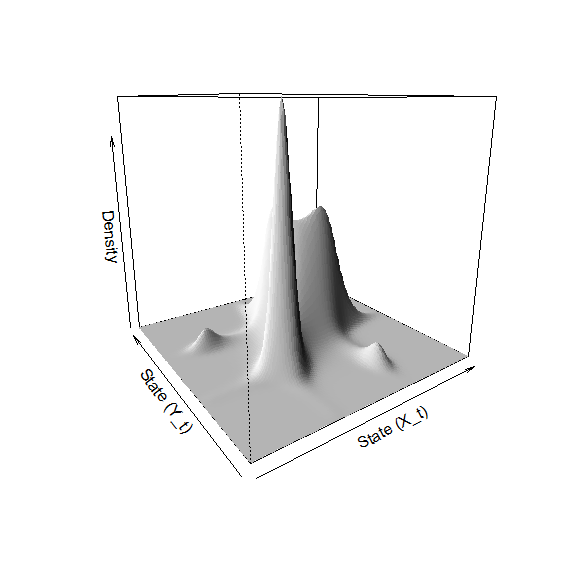# Further reading

• Related packages:
• More Vignettes:
browseVignettes('DiffusionRimp')